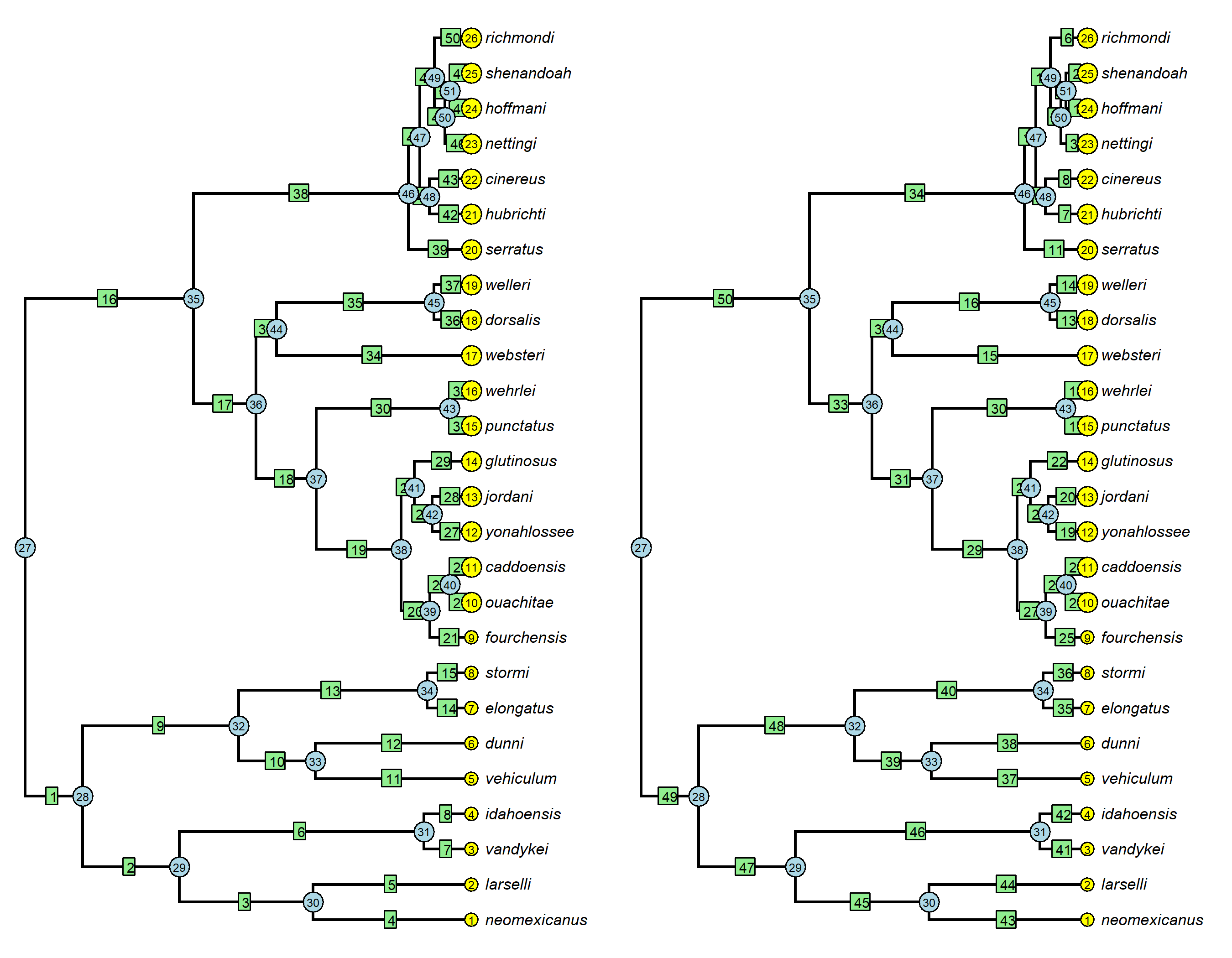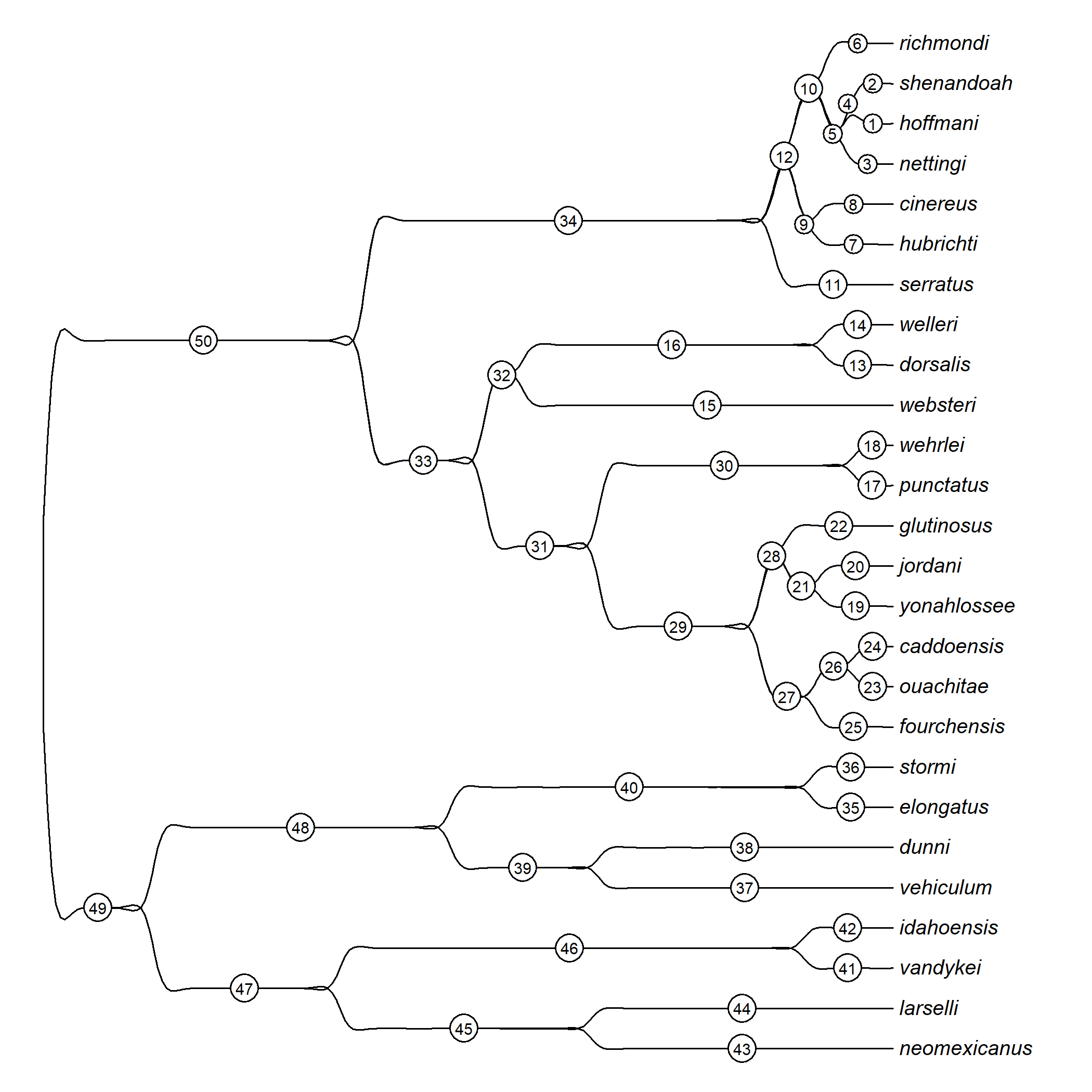## Monday, April 24, 2023

### Updated behavior of `edgelabels` for reordered trees plotted using phytools

A phytools blog reader recently pointed out that the ape function `edgelabels` did not work properly when called on a plot created using some phytools functions – if the input tree for the plot function had not been supplied in (which ape calls) “cladewise” order. (Cladewise order is an edge ordering that results from reading trees from file and makes for efficient pre-order, that is, root-to-tip, tree traversal.)

In particular, `edgelabels` assumes that the edge order matches the input tree, even though the tree has to be reordered to be plotted. (This doesn’t affect, e.g., `nodelabels` and `tiplabels`, because in that case the order is numerical by node or tip index.)

I just pushed an update to fix this. I also added a few changes so that tree plotters, such as `splinePhylogram`, that previously simply didn’t support `edgelabels` (and `nodelabels`) now do.

Let’s see.

``````library(phytools)
``````
``````data(salamanders)
par(mfrow=c(1,2))
plotTree(salamanders,ftype="i",fsize=0.7,offset=0.5)
edgelabels(cex=0.6)
nodelabels(cex=0.5,frame="circle")
tiplabels(cex=0.5,frame="circle")
postorder<-reorder(salamanders,"postorder")
plotTree(postorder,ftype="i",fsize=0.7,offset=0.5)
edgelabels(cex=0.6)
nodelabels(cex=0.5,frame="circle")
tiplabels(cex=0.5,frame="circle")
``````This difference is most easily understood by looking at the `edge` matrix of each phylogeny – here just at the first few rows.

``````head(salamanders\$edge)
``````
``````##      [,1] [,2]
## [1,]   27   28
## [2,]   28   29
## [3,]   29   30
## [4,]   30    1
## [5,]   30    2
## [6,]   29   31
``````
``````head(postorder\$edge)
``````
``````##      [,1] [,2]
## [1,]   51   24
## [2,]   51   25
## [3,]   50   23
## [4,]   50   51
## [5,]   49   50
## [6,]   49   26
``````

We see that in the first matrix, the edge connecting nodes 27 & 28 is first, 28 & 29 second, etc.; whereas, by contrast, in the second plotted tree the first two edges connect nodes 51 to the tips numbered 24 (hoffmani, in the tree) and 25 (shenandoah). Our `edgelabels` call now reflects this.

I should probably add that this kind of discrepancy is probably very rare. Outside of R phylogenetic programming, I’d say it’s quite unusual to be working with a reordered `"phylo"` object directly.

Lastly, as noted, I added node & edge labeling functionality to some plotting methods in which it was previously lacking, including `splinePhylogram`. For instance:

``````splinePhylogram(reorder(salamanders,"postorder"),ftype="i",
fsize=0.8)
edgelabels(cex=0.6,frame="circle",bg="white")
``````